Inertia & Mass Calculator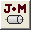The Inertia & Mass Calculator creates a table of elements and calculates the total inertia and mass. All values are saved in the project file.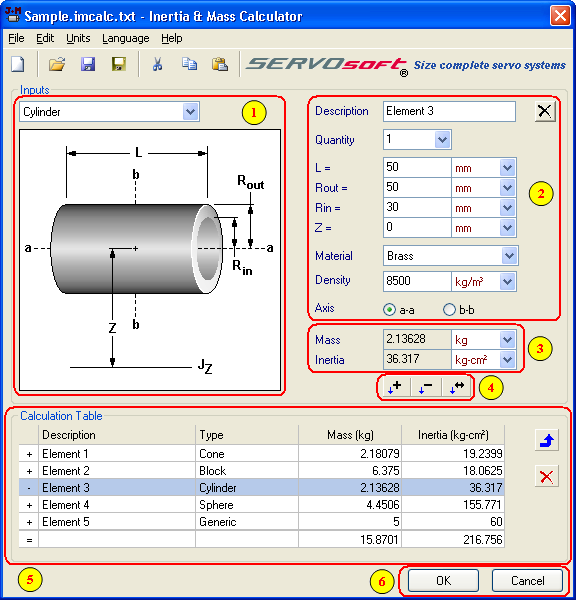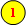Specify the element shape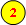Specify the inputs for a single element.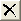Clear Inputs - Clears the single element inputs.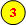The mass and inertia results for the single element.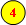New element mass and inertia added to total.New element mass and inertia subtracted from total.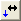Change the selected element in the calculation table with the current single element.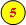The calculation table lists all elements that are added to or subtracted from the inertia and mass totals. The inertia and mass totals are applied when the OK button is selected.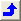Edit Selected Element - Copies the selected element in the calculation table to the single element inputs.        Tip: Double-clicking an element in the calculation table has the same effect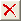Delete Selected Element - Deletes the selected element from the calculation table.When the OK button is clicked, the total inertia and mass values are copied to the clipboard, then the program ends. When the Cancel buton is clicked, the program ends.